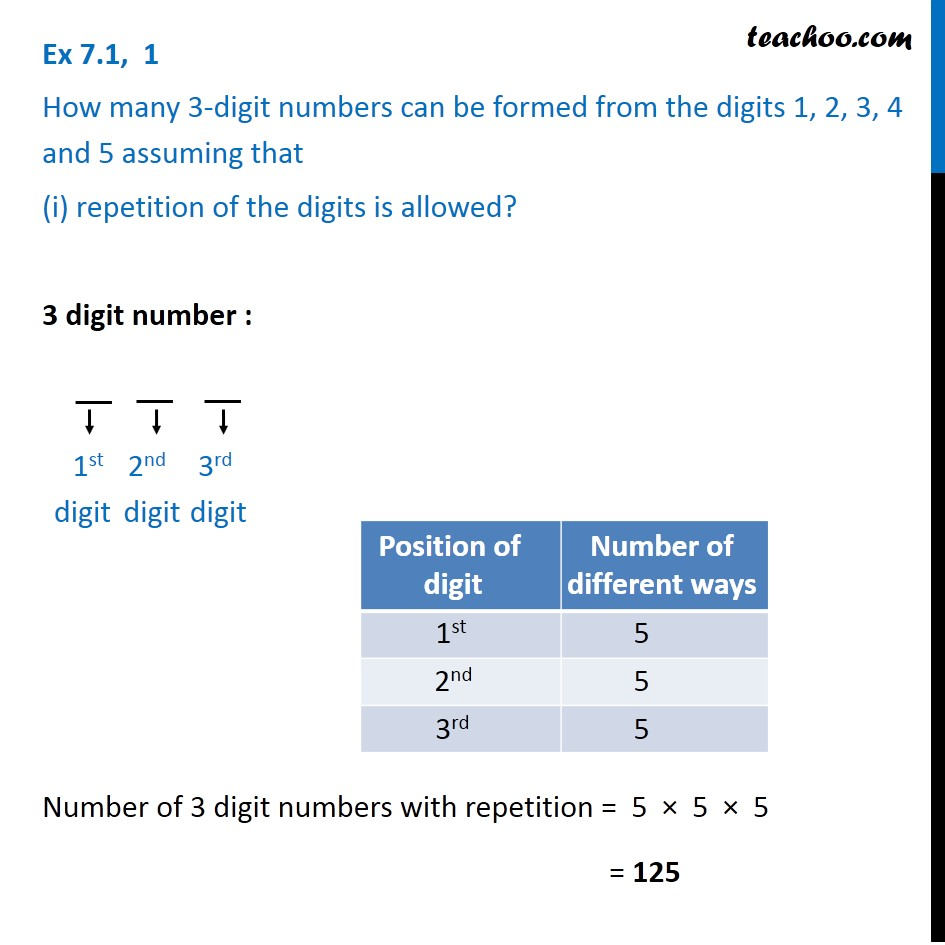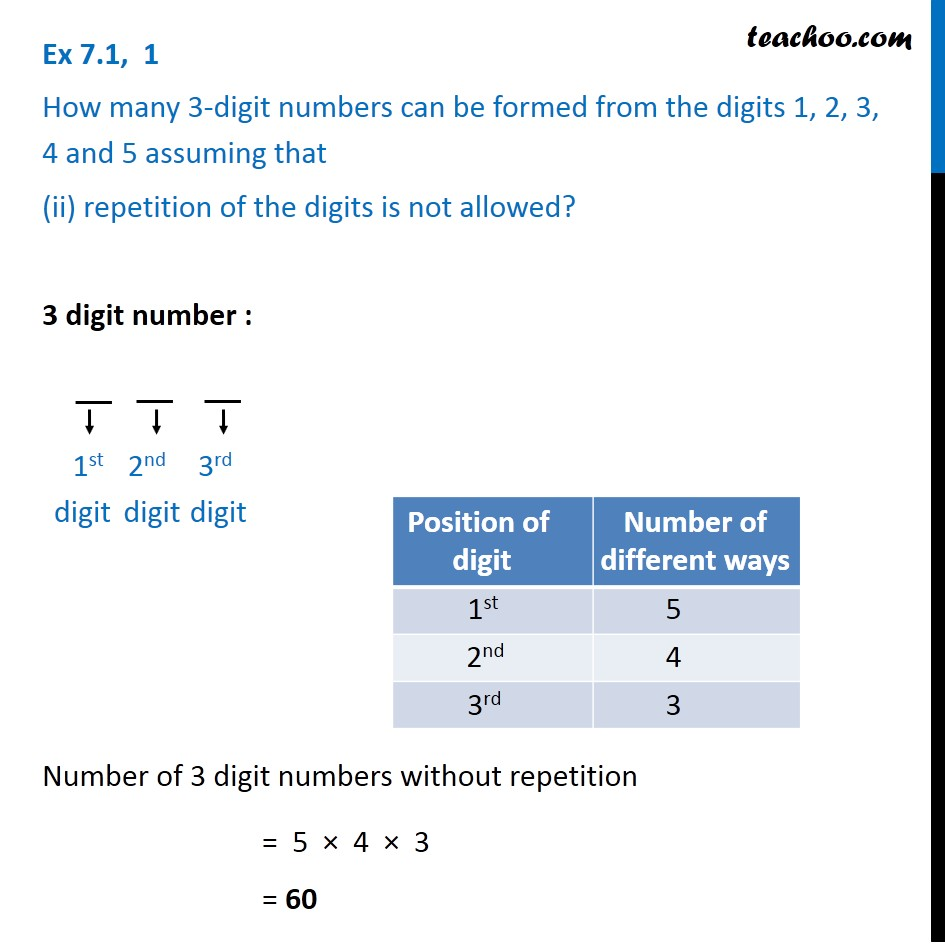Subscribe to our Youtube Channel - https://you.tube/teachoo

1. Chapter 7 Class 11 Permutations and Combinations
2. Serial order wise
3. Ex 7.1

Transcript

Ex 7.1, 1 How many 3-digit numbers can be formed from the digits 1, 2, 3, 4 and 5 assuming that (i) repetition of the digits is allowed? 3 digit number : Number of 3 digit numbers with repetition = 5 × 5 × 5 = 125 Ex 7.1, 1 How many 3-digit numbers can be formed from the digits 1, 2, 3, 4 and 5 assuming that (ii) repetition of the digits is not allowed? 3 digit number : Number of 3 digit numbers without repetition = 5 × 4 × 3 = 60 1st digit 2nd digit 3rd digit

Ex 7.1